Courses

# RD Sharma Solutions Ex-14.3, Quadrilaterals, Class 9, Maths Class 9 Notes | EduRev

## Class 9 : RD Sharma Solutions Ex-14.3, Quadrilaterals, Class 9, Maths Class 9 Notes | EduRev

The document RD Sharma Solutions Ex-14.3, Quadrilaterals, Class 9, Maths Class 9 Notes | EduRev is a part of the Class 9 Course RD Sharma Solutions for Class 9 Mathematics.
All you need of Class 9 at this link: Class 9

Q.1. In a parallelogram ABCD, determine the sum of angles ∠C and ∠D.

Solution: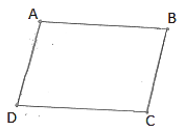∠C and ∠D are consecutive interior angles on the same side of the transversal CD.

∴∠C+∠D = 1800

Q.2. In a parallelogram ABCD, if ∠B = 1350, determine the measures of its other angles.

Solution:

Given ∠B = 1350

ABCD is a parallelogram

∴∠A = ∠C,∠B =∠Dand∠A+∠B = 1800

⇒∠A+135= 1800

⇒∠A = 450

⇒∠A = ∠C = 450 and ∠B = ∠C = 1350

Q.3. ABCD is a square. AC and BD intersect at O. State the measure of ∠AOB.

Solution:Since, diagonals of a square bisect each other at right angle.

∴ ∠AOB = 900

Q.4. ABCD is a rectangle with ∠ABD = 400. Determine ∠DBC

Solution: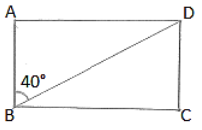We have,

∠ABC = 900

⇒∠ABD+∠DBC = 900    [∵∠ABD = 400]

⇒400+∠DBC = 900

∴∠DBC = 500

Q.5. The sides AB and CD of a parallelogram ABCD are bisected at E and F. Prove that EBFD is a parallelogram.

Solution: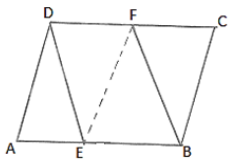Since ABCD is a parallelogram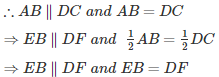EBFD is a parallelogram.

Q.6. P and Q are the points of trisection of the diagonal BD of a parallelogram ABCD. Prove that CQ is parallel to AP. Prove also that AC bisects PQ.

Solution: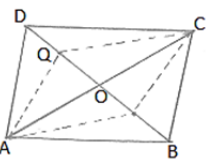We know that,

Diagonals of a parallelogram bisect each other.

Therefore, OA = OC and OB = OD

Since P and Q are point of intersection of BD.

Therefore, BP = PQ = QD

Now, OB = OD are BP = QD

⇒ OB – BP = OD – QD

⇒ OP = OQ

Thus in quadrilateral APCQ, we have

OA = OC and OP = OQ

Diagonals of Quadrilateral APCQ bisect each other.

Therefore APCQ is a parallelogram.

Hence AP∥CQ.

Q.7. ABCD is a square. E, F, G and H are points on AB, BC, CD and DA respectively, such that AE = BF = CG = DH. Prove that EFGH is a square.

Solution: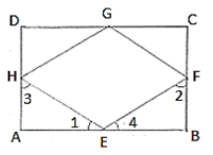We have,

AE = BF = CG = DH = x (say)

BE = CF = DG = AH = y (say)

In ΔAEH and ΔBEF,we have

AE = BF

∠A=∠B

And AH = BE

So, by SAS congruency criterion, we have

ΔAEH≃ΔBFE

⇒∠1 = ∠2 and ∠3 = ∠4

But ∠1+∠3 = 900 and ∠2+∠A = 900

⇒ ∠1+∠3+∠2+∠A = 900+900

⇒ ∠1+∠4+∠1+∠4 = 1800

⇒ 2(∠1+∠4) = 1800

⇒ ∠1+∠4 = 900

HEF = 900

Similarly we have ∠F = ∠G = ∠H = 900

Hence, EFGH is a Square.

Q.8. ABCD is a rhombus, EAFB is a straight line such that EA = AB = BF. Prove that ED and FC when produced meet at right angles.

Solution: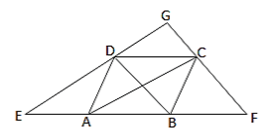We know that the diagonals of a rhombus are perpendicular bisector of each other.

∴OA = OC,OB = OD,and∠AOD = ∠COD = 900

And ∠AOB = ∠COB = 900

In ΔBDE, A and O are mid-points of BE and BD respectively.

OA∥DE

OC∥DG

In ΔCFA, B and O are mid-points of AF and AC respectively.

OB∥CF

OD∥GC

Thus, in quadrilateral DOGC, we have

OC∥DGandOD∥GC

⇒ DOCG is a parallelogram

∠DGC = ∠DOC

∠DGC = 900

Q.9. ABCD is a parallelogram, AD is produced to E so that DE = DC and EC produced meets AB produced in F. Prove that BF = BC.

Solution:

Draw a parallelogram ABCD with AC and BD intersecting at O.

Produce AD to E such that DE = DC

Join EC and produce it to meet AB produced at F.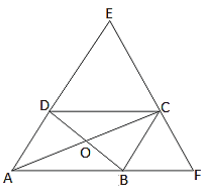In ΔDCE,

∠DCE =∠DEC…..(i)     [In a triangle, equal sides have equal angles]

AB∥CD              [Opposite sides of the parallelogram are parallel]

∴AE∥CD              [AB lies on AF]

AF∥CD and EF is the Transversal.

∠DCE = ∠BFC…..(ii)          [Pair of corresponding angles]

From (i) and (ii) we get

∠DEC = ∠BFC

In ΔAFE,

∠AFE = ∠AEF                    [∠ DEC = ∠ BFC]

Therefore, AE = AF          [In a triangle, equal angles have equal sides opposite to them]

⇒ AD + DE = AB + BF

⇒BC + AB = AB + BF          [Since, AD = BC, DE = CD and CD = AB, AB = DE]

⇒ BC = BF

Hence proved.

Offer running on EduRev: Apply code STAYHOME200 to get INR 200 off on our premium plan EduRev Infinity!

91 docs

,

,

,

,

,

,

,

,

,

,

,

,

,

,

,

,

,

,

,

,

,

,

,

,

,

,

,

,

,

,

;